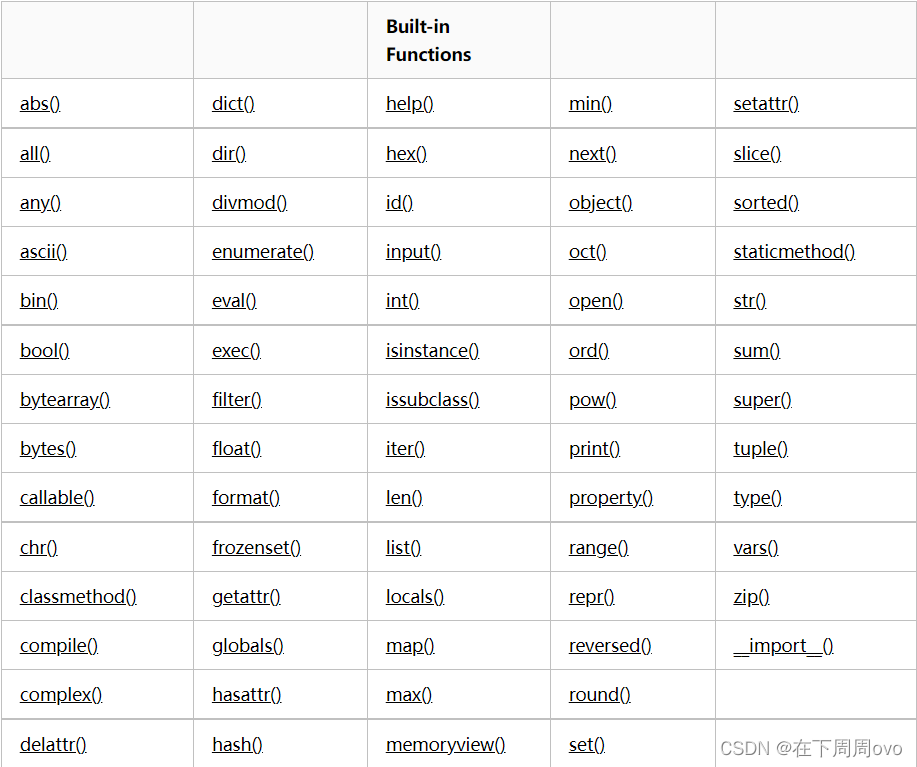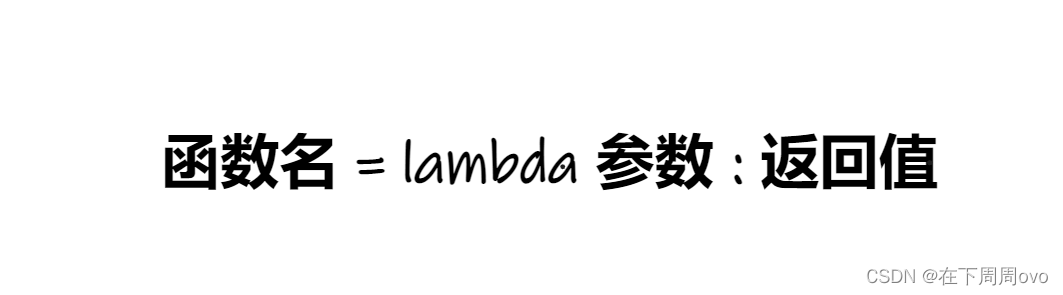# 🏵️前言

🍁一、reversed内置函数

🍁二、slice内置函数

🍁三、format内置函数

🍁四、bytes内置函数

🍁五、bytearray内置函数

🍁六、memoryview内置函数

🍁七、ord、chr和ascii内置函数

🍁八、repr内置函数

🍁九、enumerate内置函数

🍁十、all和any内置函数

🍁十一、zip内置函数

🍁十二、filter和map内置函数

🔥1、filter内置函数

🔥2、map内置函数

🔥3、总结

🍁十三、sorted内置函数

🍁十四、匿名函数

# 🏵️前言## 🍁一、reversed内置函数

reversed 函数返回一个反转的迭代器。

reversed(seq)

seq — 要转换的序列，可以是 tuple, string, list 或 range。

``````#使用reverse后原列表就不见了
l = [1,2,3,4,5]
l.reverse()
print(l)

#保留原列表，返回一个反向的迭代器
l = [1,2,3,4,5]
l2 =  reversed(l)
print(l2)

[5, 4, 3, 2, 1]
<list_reverseiterator object at 0x000001BF41858F40>``````

## 🍁二、slice内置函数

slice() 函数实现切片对象，主要用在切片操作函数里的参数传递。

slice 语法：

class slice(stop)
class slice(start, stop[, step])

start — 起始位置
stop — 结束位置
step — 间距

``````l = (1,2,3,4,5,6)
#先得到了一个切片规则
sli = slice(1,5,2)
#按切片规则进行切分
print(l[sli])

(2, 4)``````

## 🍁四、bytes内置函数

bytes 函数返回一个新的 bytes 对象，该对象是一个 0 <= x < 256 区间内的整数不可变序列。它是 bytearray 的不可变版本。

class bytes([source[, encoding[, errors]]])

html网页爬取到的也是编码

``````#我拿到的是gbk编码的，我想转成utf-8编码
print(bytes('你好',encoding='gbk').decode('gbk'))
#unicode转换为utf-8的bytes
print(bytes('你好',encoding='utf-8'))

b'\xe4\xbd\xa0\xe5\xa5\xbd'``````

## 🍁五、bytearray内置函数

bytearray() 方法返回一个新字节数组。这个数组里的元素是可变的，并且每个元素的值范围: 0 <= x < 256。

bytearray()方法语法：

class bytearray([source[, encoding[, errors]]])

``````b_array = bytearray('你好',encoding='utf-8')
print(b_array)
print(b_array)

bytearray(b'\xe4\xbd\xa0\xe5\xa5\xbd')
228``````

## 🍁六、memoryview内置函数

memoryview() 函数返回给定参数的内存查看对象(memory view)。

memoryview(obj)

obj — 对象

``````#切片 —— 字节类型的切片
v = memoryview(bytearray("abcd", 'utf-8'))
print(v)

98``````

## 🍁七、ord、chr和ascii内置函数

``````#ord     将字符按照unicode转数字
>>>print(ord('a'))
>>>print(ord('A'))
>>>print(ord('1'))
97
65
49

#chr     数字按照unicode转字符
>>>print(chr(65))
>>>print(chr(97))
A
a

#ascii   只要是ASCII码中的内容就打印出来，不是就转换为\u
#ascii   包括字母、数字、符号、拉丁文
>>>print(ascii('你好'))
>>>print(ascii(1))
>>>print(ascii("_12"))
>>>print(ascii('a'))
'\u4f60\u597d'
1
'_12'
'a'``````

## 🍁八、repr内置函数

repr() 函数将对象转化为供解释器读取的形式。

repr(object)

object — 对象。

``````#可以判断控制台输出的数据是何类型
print(repr('1'))
print(repr(1))

'1'
1``````

## 🍁九、enumerate内置函数

enumerate() 函数用于将一个可遍历的数据对象(如列表、元组或字符串)组合为一个索引序列，同时列出数据和数据下标，一般用在 for 循环当中。

enumerate(sequence, [start=0])

sequence — 一个序列、迭代器或其他支持迭代对象。
start — 下标起始位置。

``````lst = ['a','b','c']
print(list(enumerate(lst)))
for i in enumerate(lst):
print(i)

[(0, 'a'), (1, 'b'), (2, 'c')]
(0, 'a')
(1, 'b')
(2, 'c')``````

## 🍁十、all和any内置函数

• all：用于判断给定的可迭代参数 iterable 中的所有元素是否有bool值为False，如果有则返回 True，否则返回 False。元素除了是 0、空、None、False 外都算 True。
• any：用于判断给定的可迭代参数 iterable 是否有bool值为 True的元素，如果有一个为 True，没有一个则返回 False。元素除了是 0、空、FALSE 外都算 TRUE。
• ``````print(all([1,'','a']))
print(all([1,'a']))
print(all([0,123]))
输出结果：
False
True
False

print(any(['','Ture',123]))
print(any(['',0]))
输出结果：
True
False``````

## 🍁十一、zip内置函数

描述
zip() 函数用于将可迭代的对象作为参数，将对象中对应的元素打包成一个个元组，然后返回由这些元组组成的对象，这样做的好处是节约了不少的内存。

我们可以使用 list() 转换来输出列表。

如果各个迭代器的元素个数不一致，则返回列表长度与最短的对象相同，利用 * 号操作符，可以将元组解压为列表。

zip 方法在 Python 2 和 Python 3 中的不同：在 Python 2.x zip() 返回的是一个列表。

语法
zip 语法：

zip([iterable, …])
参数说明：

iterabl — 一个或多个迭代器;
返回值
返回一个对象。

``````l1 = [1,2,3]
l2 = ['a','b','c','d']
l3 = [{1,2},'**']
print(zip(l1,l2))
for i in zip(l1,l2):
print(i)
print("=======================")
for i in zip(l1,l2,l3):
print(i)

输出结果：
<zip object at 0x000001967CE0B600>
(1, 'a')
(2, 'b')
(3, 'c')
=======================
(1, 'a', {1, 2})
(2, 'b', '**')
``````

## 🍁十二、filter和map内置函数

### 🔥1、filter内置函数

filter() 函数用于过滤序列，过滤掉不符合条件的元素，返回一个迭代器对象，如果要转换为列表，可以使用 list() 来转换。

该接收两个参数，第一个为函数，第二个为序列，序列的每个元素作为参数传递给函数进行判断，然后返回 True 或 False，最后将返回 True 的元素放到新列表中。

``````def is_odd(x):
return x % 2 == 1

ret = filter(is_odd,[1,2,4,7,9])
print(ret)
print([i for i in ret])

输出结果：
<filter object at 0x000001FF38B48640>
[1, 7, 9]
``````

### 🔥2、map内置函数

map() 函数会根据提供的函数对指定序列做映射。

第一个参数 function 以参数序列中的每一个元素调用 function 函数，返回包含每次 function 函数返回值的新列表。

``````ret = map(abs,[1,-4,6,-9])
print(ret)
print([i for i in ret])

输出结果：
<map object at 0x000001CF1D7C90A0>
[1, 4, 6, 9]``````

### 🔥3、总结

filter  执行了filter之后的结果集合元素个数 <= 执行之前的个数
filter只管筛选，执行前后不会改变原来的值
map     执行前后元素个数保持不变
map执行前后原来的值可能会发生改变

## 🍁十四、匿名函数

匿名函数：为了解决那些功能很简单的需求而设计的一句话函数``````#将以下函数变为匿名函数
return x + y

add2 = lambda x,y : x + y